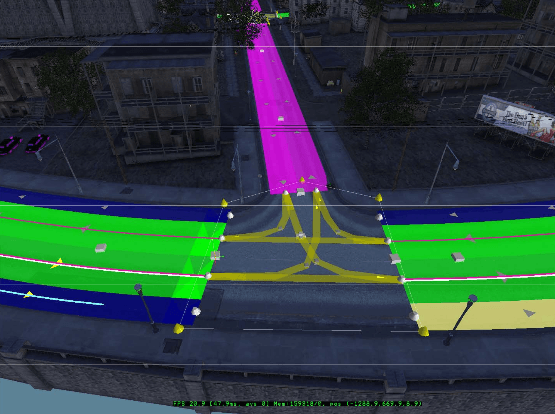# Everything about interpolation in Unity with C# code

## 1. Generate a Catmull-Rom spline

Here you will create your very own Catmull-Rom spline in Unity. The name orignates from the creators of the spline: Edwin Catmull, co-founder of Pixar, and Raphael Rom. So the Catmull-Rom splines are often used in 3D animations. But the splines are also very popular to use in computer games. For example, the game Mafia uses Catmull-Rom splines to connect waypoints.Catmull-Rom splines in Mafia. Source

In this tutorial we will focus on the very basics. We will create a smooth path between a series of spheres. And if we want to create a connected path so it can form a loop, we will also be able to do that. This is the beauty of the Catmull-Rom spline: if you have a series of points and want to generate a smooth curve along these points, it's possible. The reason is that the curve will use all points to form the curve (except the first and last point if you are not making a loop). Compare this with the Bezier curve, which will need extra control points which are not a part of the spline itself.

What's the basic idea behind the Catmull-Rom spline?. The answer is a cubic polynomial, which looks like this: f(t) = a_3 * t^3 + a_2 * t^2 + a_1 * t + a_0. It's your job to determine the coefficients a. To help, you can use this link.

If you have 4 points called p0, p1, p2, and p3, you will be able to add a smooth path between the 2 middle points p1 and p2 (if the curve is not forming a loop). The middle points are called the curve´s endpoints. We need the first and last point (p0 and p3) to define the curve's control points that control the shape of the curve.

To create the curve we will move from p1 to p2 with a parameter called t (the same t as is in the cubic polynomial above). This t will always be a number between 0 and 1. If t is 0 we are exactly at the same coordinate as p1, and if t is 1 we are exactly at the same coordinate as p2. This link will explain it better if you are lost.

What you first have to do after you have created a new scene in Unity is to create an empty GameObject. As children to that GameObject, you should create 4 spheres. Name these something like p1, p2, p3,... because they will form our path. Remember to create at least 4 of them, because that is the minimum number of points in a Catmull-Rom spline. Then create a new script called CatmullRomSpline, and add the following:

```using UnityEngine;
using System.Collections;

//Interpolation between points with a Catmull-Rom spline
public class CatmullRomSpline : MonoBehaviour
{
//Has to be at least 4 points
public Transform[] controlPointsList;
//Are we making a line or a loop?
public bool isLooping = true;

//Display without having to press play
void OnDrawGizmos()
{
Gizmos.color = Color.white;

//Draw the Catmull-Rom spline between the points
for (int i = 0; i < controlPointsList.Length; i++)
{
//Cant draw between the endpoints
//Neither do we need to draw from the second to the last endpoint
//...if we are not making a looping line
if ((i == 0 || i == controlPointsList.Length - 2 || i == controlPointsList.Length - 1) && !isLooping)
{
continue;
}

DisplayCatmullRomSpline(i);
}
}

//Display a spline between 2 points derived with the Catmull-Rom spline algorithm
void DisplayCatmullRomSpline(int pos)
{
//The 4 points we need to form a spline between p1 and p2
Vector3 p0 = controlPointsList[ClampListPos(pos - 1)].position;
Vector3 p1 = controlPointsList[pos].position;
Vector3 p2 = controlPointsList[ClampListPos(pos + 1)].position;
Vector3 p3 = controlPointsList[ClampListPos(pos + 2)].position;

//The start position of the line
Vector3 lastPos = p1;

//The spline's resolution
//Make sure it's is adding up to 1, so 0.3 will give a gap, but 0.2 will work
float resolution = 0.2f;

//How many times should we loop?
int loops = Mathf.FloorToInt(1f / resolution);

for (int i = 1; i <= loops; i++)
{
//Which t position are we at?
float t = i * resolution;

//Find the coordinate between the end points with a Catmull-Rom spline
Vector3 newPos = GetCatmullRomPosition(t, p0, p1, p2, p3);

//Draw this line segment
Gizmos.DrawLine(lastPos, newPos);

//Save this pos so we can draw the next line segment
lastPos = newPos;
}
}

//Clamp the list positions to allow looping
int ClampListPos(int pos)
{
if (pos < 0)
{
pos = controlPointsList.Length - 1;
}

if (pos > controlPointsList.Length)
{
pos = 1;
}
else if (pos > controlPointsList.Length - 1)
{
pos = 0;
}

return pos;
}

//Returns a position between 4 Vector3 with Catmull-Rom spline algorithm
//http://www.iquilezles.org/www/articles/minispline/minispline.htm
Vector3 GetCatmullRomPosition(float t, Vector3 p0, Vector3 p1, Vector3 p2, Vector3 p3)
{
//The coefficients of the cubic polynomial (except the 0.5f * which I added later for performance)
Vector3 a = 2f * p1;
Vector3 b = p2 - p0;
Vector3 c = 2f * p0 - 5f * p1 + 4f * p2 - p3;
Vector3 d = -p0 + 3f * p1 - 3f * p2 + p3;

//The cubic polynomial: a + b * t + c * t^2 + d * t^3
Vector3 pos = 0.5f * (a + (b * t) + (c * t * t) + (d * t * t * t));

return pos;
}
}
```

That wasn't too difficult? If you go to the editor you should be able to do this: